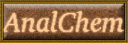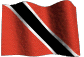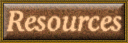# Volumetric Analysis Primary Standard solutions.

Search this site

## How to make up 250ml 0.05M and 0.1N Volumetric analysis Primary Standards

This page gives the weight of pure, dry salt required to make up a 250 ml volumetric analysis primary standard for each titration against acid base, permanganate, dichromate, iodine, sodium thiosulphate and silver nitrate.
Concentrations are expressed in normality or molarity and given in values of 0.1N, 0.05M, or 0.002M.

Example for the info on this page:

 Sodium carbonate Na2CO3, FW = 106, 1M = 106g/l Eq.wt. = 53g/l = 1N = 0.5M 1 liter = 5.30g/l = 0.1N = 0.05M 250ml = 1.325g = 0.IN or 0.05M std. soln.Dissolve 1.325g of the salt in 250ml volumetric flask to give a 0.05M or a 0.1N equivalent std soln.

 In this example, the FW or Formula weight (Molecular weight) of the salt is 106. Eq.wt. is the Equivalent Weight which is 53 grams per liter. And 1.325 grams (5.30 g/l divided by 4) dissolved in 250 ml volumetric flask will give a 0.1N standard solution or a 0.05M standard solution.

## Acid / Base Titrations

 Ammonium sulphate NH4)2SO4. FW = 132.14, 1M = 132.14g/l Eq.wt. = 66.07g/l = 1N = 0.5M 1 liter = 6.607g/l = 0.1N =0.05M 250ml = 1.652g = 0.IN or 0.05M std. soln.Dissolve 1.652g of the salt in 250ml volumetric flask to give a 0.05M or a 0.1N equivalent std soln. Adipic acid HO2C(CH2)4CO2H FW = 146.4, 1M = 146.4g/l Eq.wt. = 73.03g/l =1N = 0.5M 1 liter = 7.303g/l = 0.1N = 0.05M 250ml = 1.83g = 0.1N or 0.05M std. soln.Dissolve 1.83g of the salt in 250ml volumetric flask to give a 0.05M or a 0.1N equivalent std soln. Barium hydroxide Ba(OH)2.8H2O, FW = 315.48, 1M = 315.48g/l Eq.wt. = 157.5g/l = 1N or 0.5M 1 liter = 15.75g/l = 0.1N = 0.05M 250ml = 3.94g =0.1N or 0.05M std. soln.Dissolve 3.94g of the salt in 250ml volumetric flask to give a 0.05M or a 0.1N equivalent std soln. Benzoic acid C6H5COOH, FW = 122.12, 1M = 122.12g/l Eq.wt. = 61.06g/l = 1N = 0.5M 1 liter = 6.106g/l = 0.1N = 0.05M 250ml = 1.52g = 0.1N or 0.05M std. soln.Dissolve 1.52g of the salt in 250ml volumetric flask to give a 0.05M or a 0.1N equivalent std soln. Calcium carbonate CaCO3, FW = 100.00, 1M = 100g/l Eq.wt. = 50g/l = 1N = 0.5M 1 liter = 5.0g/l = 0.1N =0.05M 250ml = 1.25g = 0.IN or 0.05M std. soln.Dissolve 1.25g of the salt in 250ml volumetric flask to give a 0.05M or a 0.1N equivalent std soln. Furroic acid FW = 112.08, 1M = 112.08g/l Eq.wt. = 112.08 = 1N = 1M 1 liter = 11.2g/l = 0.1N =0.1M 250ml = 2.8g = 0.IN or 0.1M std. soln.Dissolve 2'8g of the salt in 250ml volumetric flask to give a 0.1M or a 0.1N equivalent std soln. Hydrochloric acid HCl, FW = 36.5, Density = 1.2 1M = 83mls = 1N = 1M (Use 86mls) 250ml = 2mls = 0.1N = 0.1N 1 liter = 8.6mls = 0.1N or 0.1M soln.Measure 2mls of the acid in 250ml volumetric flask to give a 0.1M or a 0.1N equivalent soln. Oxalic acid H2C2O4.2H2O, FW = 126.07, 1M = 126.07g/l Eq.wt. = 63.03g/l = 1N = 0.5M 1 liter = 6.303g/l = 0.1N =0.05M 250ml = 1.575g = 0.IN or 0.05M std. soln.Dissolve 1.575g of the salt in 250ml volumetric flask to give a 0.05M or a 0.1N equivalent std soln. Potassium hydrogen phthalate KH(C8H4O4), FW = 204.23, 1M 204.23g/l Eq.wt. = 204.23g/l = 1N = 1M 1 liter = 204.23g/l = 0.1N =0.1M 250ml = 5.105g = 0.IN or 0.1M std. soln.Dissolve 5.105g of the salt in 250ml volumetric flask to give a 0.1M or a 0.1N equivalent std soln. Potassiun hydrogen iodate KH(IO3)2, FW = 389.92, 1M = 389.82g/l Eq.wt. = 77.98g/l = 1N = 0.2M 1 liter = 7.798g/l = 0.1N =0.02M 250ml = 1.95g = 0.IN or 0.02M std. soln.Dissolve 1.95g of the salt in 250ml volumetric flask to give a 0.02M or a 0.1N equivalent std soln. Sodium carbonate Na2CO3, FW = 106, 1M = 106g/l Eq.wt. = 53g/l = 1N = 0.5M 1 liter = 5.30g/l = 0.1N =0.05M 250ml = 1.325g = 0.IN or 0.05M std. soln.Dissolve 1.325g of the salt in 250ml volumetric flask to give a 0.05M or a 0.1N equivalent std soln. Sodium hydroxide NaOH, FW = 40, 1M = 40g/l Eq.wt. = 40.00g/l = 1N = 1M 1 liter = 4.0g/l = 0.1N =0.1M 250ml = 1.00g = 0.IN or 0.1M std. soln.Dissolve 1.00g of the salt in 250ml volumetric flask to give a 0.1M or a 0.1N equivalent soln. Sodium oxalate Na2C2O4, FW = 134.00, 1M = 134g/l Eq.wt. = 134g/l = 1N = 1M 1 liter = 13.40g/l = 0.1N = 0.1M 250ml = 3.35g = 0.IN or 0.1M std. soln.Dissolve 3.35g of the salt in 250ml volumetric flask to give a 0.1M or a 0.1N equivalent std soln. Sodium tetraborate NaB4O7.10H2O, FW = 381.37, 1M = 381.37g/l Eq.wt. = 190.685g/l = 1N = 0.5M 1 liter = 190.68g/l = 0.1N = 0.05M 250ml = 4.767g = 0.IN or 0.05M std. soln.Dissolve 4.767g of the salt in 250ml volumetric flask to give a 0.05M or a 0.1N equivalent std soln. Succinic acid HO2CCH2CH2CO2H, FW = 118.09, 1M = 118.09g/l Eq.wt. = 59.045g/l = 1N = 0.5M 1 liter = 5.9.045g/l = 0.1N = 0.05M 250ml = 1.475g = 0.IN or 0.05M std. soln.Dissolve 1.475g of the salt in 250ml volumetric flask to give a 0.05M or a 0.1N equivalent std soln. Sulphamic acid H2NSO3H, FW = 97.09, 1M = 97.09g/l Eq.wt. = 97.09g/l = 1N = 1M 1 liter = 9.709g/l = 0.1N =0.1M 250ml = 2.427g = 0.IN or 0.1M std. soln.Dissolve 2.427g of the salt in 250ml volumetric flask to give a 0.1M or a 0.1N equivalent std soln. Sulphuric acid H2SO4, FW = 98.08, Density = 1.8 1 liter = 56mls = 2N = 1M 1 liter = 28mls = 1N = 0.05M 1 liter = 2.8mls = 0.1N or 0.05M (use 3 mls) 250ml = 0.7mls = 0.1N = 0.05M std.Measure 0.7mls of the acid in 250ml volumetric flask to give a 0.05M or a 0.1N equivalent soln. Tris (hydroxymethyl)-aminomethane H2N.C(CH2OH)3, FW = 121.14, 1M = 121.14g/l Eq.wt. = 121.14g/l = 1N = 1M 1 liter = 12.114g/l = 0.1N = 0.1M 250ml = 3.023g = 0.IN or 0.1M std. soln.Dissolve 3.023g of the salt in 250ml volumetric flask to give a 0.1M or a 0.1N equivalent std soln.